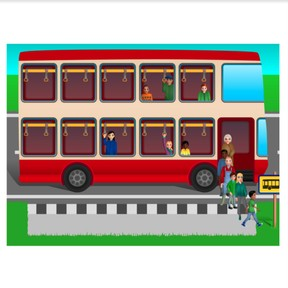On the bus: Subtraction to 20 crossing ten

# On the bus: Subtraction to 20 crossing ten

No account needed.8,000 schools use Gynzy92,000 teachers use Gynzy1,600,000 students use Gynzy

## General

Students learn to subtract numbers to 20 using a bus ride as context, crossing ten.

1.OA.C

## Relevance

To learn how to subtract well, it is important to be able to subtract to 20. Using the context of a bus ride helps make subtraction easier and faster and better understand subtraction.

## Introduction

Students are shown an image of a bus on the interactive whiteboard. Ask students who has ridden a bus recently. Ask if it was a full or empty bus. Next practice composing to 10 from a given number by asking the students how many more kids are needed to make 10.

## Development

Using the three images, explain how subtraction on the bus work. The first image shows how many passengers are on the bus as it arrives. The second shows how many passengers exit the bus at the stop. The third image shows how many passengers are left on the bus as it leaves. You can determine this third image total by taking away the number of exiting passengers from the total number of passengers from the first image. Ask students to name which number is the first number of the subtraction problem (minuend) which is the second number of the subtraction problem (subtrahend) and what is the total, or difference. Tell students that they can check their work by adding their total (difference) and second number (subtrahend) together.
Next practice two more bus examples on the interactive whiteboard. Explain to students that the images will now stay the same- you can have all the information in just one image. Practice this with two bus examples and show students that they can also solve using the bus method even when they can't count the individual passengers, but are given numbers to represent the number of passengers on the bus. Tell students that they can also split their second number (subtrahend) to make subtraction easier when you have to cross ten. Practice with the students.

To check that students are able to subtract numbers to 20 using the context of a bus ride crossing ten, you can ask the following questions:
- What are the steps to solving a subtraction problem on the bus?
- What does the first number in the subtraction problem represent?
- What does the second number in the subtraction problem represent?

## Guided practice

Students are first given a subtraction problem with three images and countable passengers on the bus. They are then asked to solve the subtraction problem with one of the numbers given without visual support and are finally asked to solve the entire subtraction problem without visual support.

## Closing

Repeat the learning goal and remind students of the steps to take when subtracting on the bus. Make groups and give each group a set of number cards to 20. They must split the number cards into two piles, one of the numbers 0 -10, and the second from 11-20. They turn over a card from the 11-20 stack. This is the number of passengers on the bus. They then turn over the card from the 0-10 stack. This is the number of passengers exiting the bus. They solve the problem. They continue through both stacks.

## Teaching tips

Students who have difficulty with this learning goal can be supported by a clear breakdown of steps. First counting the total passengers on the bus, then counting how many passengers get off the bus, and finally counting how many passengers are still left on the bus. They can use blocks or other manipulatives to represent the passengers.

## Instruction materials

Sets of number cards from 1-20.
Optional- blocks or other manipulatives

### The online teaching platform for interactive whiteboards and displays in schools

• Save time building lessons

• Manage the classroom more efficiently

• Increase student engagement